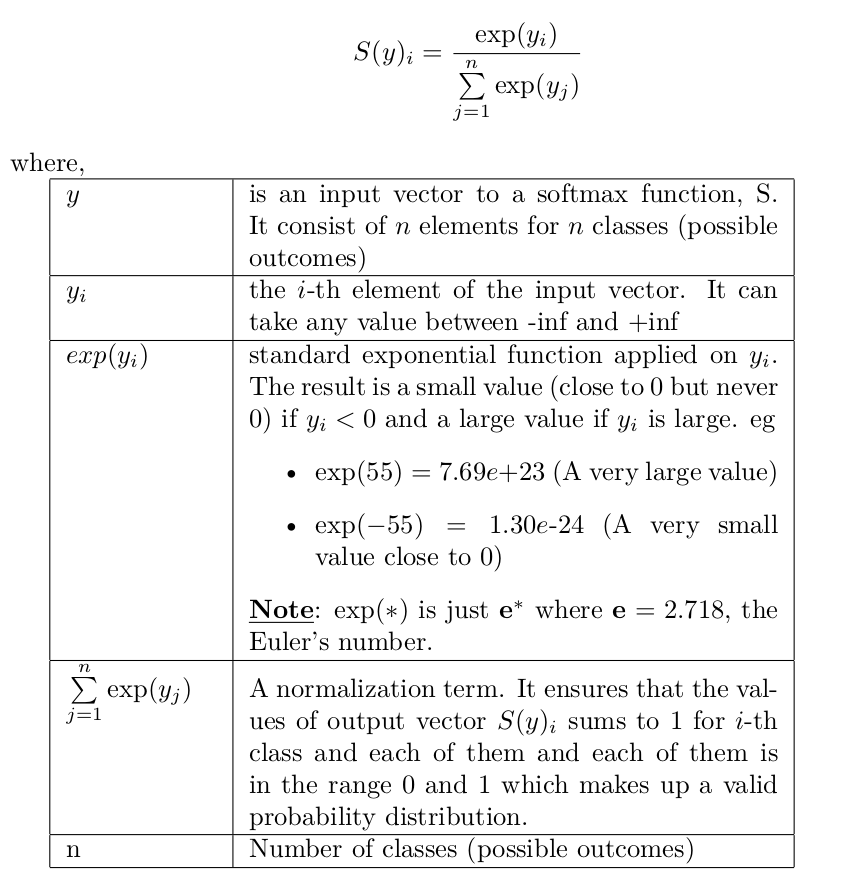# Softmax Activation Function — How It Actually WorksWhen working on machine learning problems, specifically, deep learning tasks, Softmax activation function is a popular name. Softmax is a function placed at the end of deep learning network to convert logits into classification probabilities.

When working on machine learning problems, specifically, deep learning tasks, Softmax activation function is a popular name. It is usually placed as the last layer in the deep learning model.

It is often used as the last activation function of a neural network to normalize the output of a network to a probability distribution over predicted output classes. — Wikipedia [link]

Softmax is an activation function that scales numbers/logits into probabilities. The output of a softmax is a vector (say `v`) with probabilities of each possible outcome. The probabilities in vector `v` sums to one for all possible outcomes or classes.

Mathematically, Softmax is defined as,## Cheat Sheets for AI, Neural Networks, Machine Learning, Deep Learning & Big Data

Cheat Sheets for AI, Neural Networks, Machine Learning, Deep Learning & Big Data

## Deep Learning 101 —  Neural Networks Explained

The past few decades have witnessed a massive boom in the penetration as well as the power of computation, and amidst this information.

## Most popular Data Science and Machine Learning courses — July 2020

Most popular Data Science and Machine Learning courses — August 2020. This list was last updated in August 2020 — and will be updated regularly so as to keep it relevant

## Artificial Neural Networks — Recurrent Neural Networks

Artificial Neural Networks — Recurrent Neural Networks. Remembering the history and predicting the future with neural networks. A intuition behind Recurrent neural networks.

## Deep Learning Tutorial with Python | Machine Learning with Neural Networks

In this video, Deep Learning Tutorial with Python | Machine Learning with Neural Networks Explained, Frank Kane helps de-mystify the world of deep learning and artificial neural networks with Python!